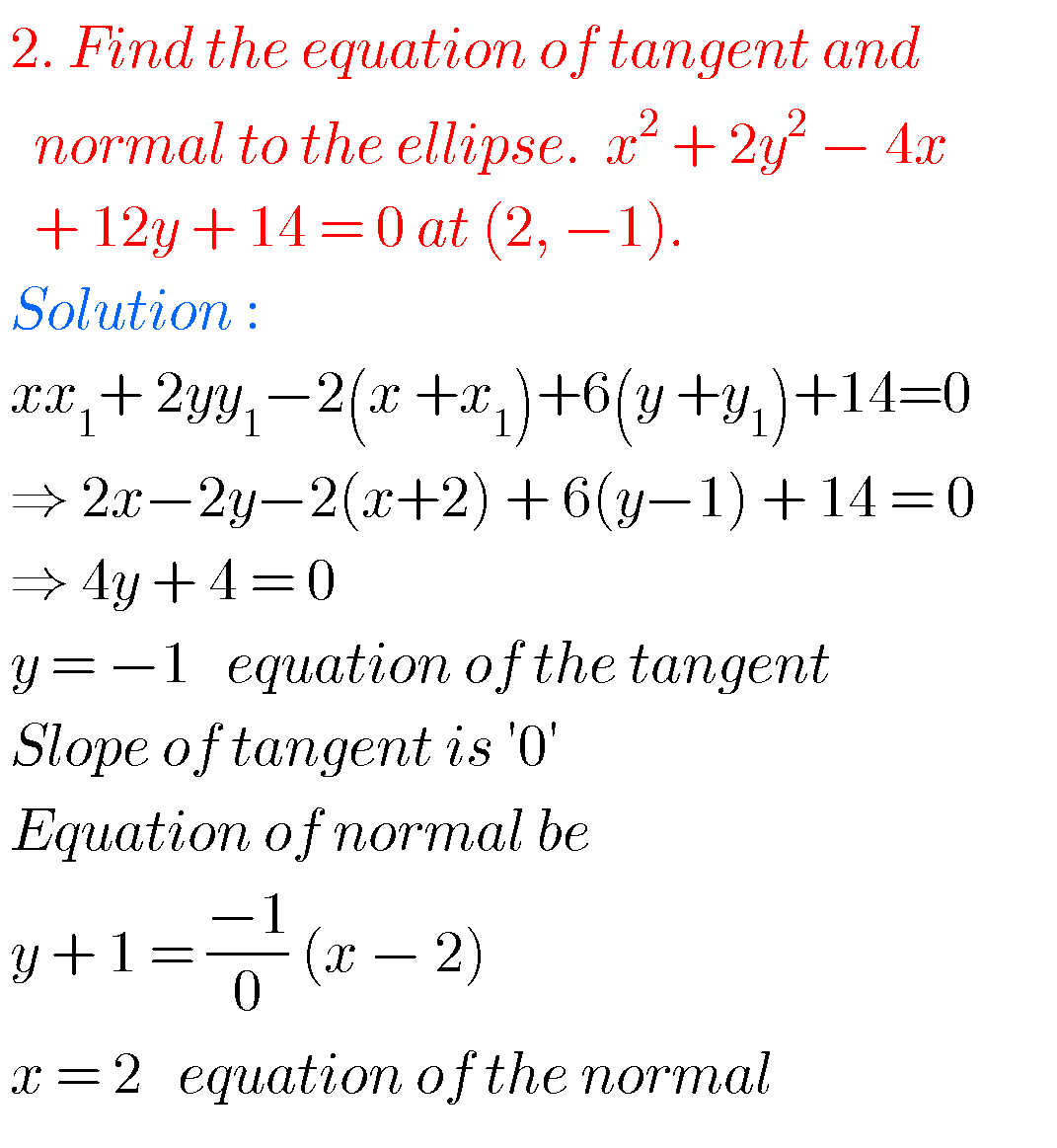## Intermediate Maths solutions for Ellipse exercise 4a & 4b

Intermediate Maths solutions for Ellipse Inter Maths IIB solutions for Ellipse exercise 4(a) and 4(b) are given. These solutions are very easy to understand. First you study the textbook lesson very well. Then you observe the example problems and solutions. Try them. Next try exercise problems. Observe the given solutions and try them in your …